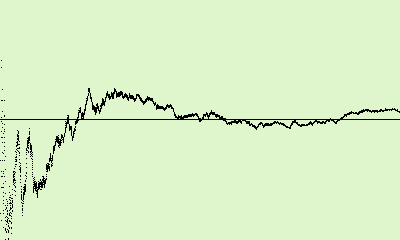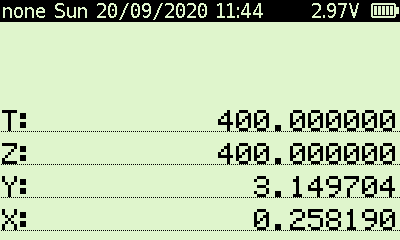### Using random numbers to compute the value of π

This is a little foray into number theory and presents one method of calculating the value of $\pi$. It involves using a program to perform iterative calculations, so in order to minimise the impact of compounded rounding errors, it makes sense to use a computing device that operates with sufficient precision. I chose the SwissMicros DM42 for this because it uses Thomas Okken's Free42 Decimal under the hood, which offers around 34 digits of precision. The DM42 is also extremely fast, particularly so if connected to a USB port while operating because it more than triples the CPU clock compared to battery-powered operation.

Back in 1735, the Swiss mathematician, astronomer (and a few other things besides) Leonhard Euler stated, and proved in 1741, that as the number $n$ tends towards $\infty$, the probability that two natural numbers $a$ and $b$ less than or equal to $n$ are coprime, i.e. that $a$ and $b$ share no common divisor other than $1$, tends towards $\frac 6{\pi^2} \approx 0.607927$ (Wikipedia).

So, what's to stop us from writing a program to sample pairs of natural numbers, count how many of them are coprime and examine the proportion of coprime pairs to the total number of pairs? I'll call this proportion that we find experimentally $p$.

$p$ should reflect the probability demonstrated by Euler and should therefore be close to our value of $\frac 6{\pi^2}$. All we have to do then is calculate $\sqrt {\frac 6p}$ and that will give us our approximation of $\pi$.

A few caveats first. Firstly, this only gets close to reality for values of $n$ (the highest random natural number that we work with) that approach $\infty$. However, we get an error of only about 0.3% if we stick to $n=200$ or about 0.06% for $n=1000$. Why? Because the probability of choosing two coprimes from the first $n$ natural numbers is: $$\frac 1{\sum_{k=1}^n \frac 1{k^2}}$$ Only if $n=\infty$ do we get: $$\frac 1{\sum_{k=1}^\infty \frac 1{k^2}} = \frac 1{\zeta(2)} = \frac 6{\pi^2}$$ Ref: "Basel" problem, Riemann Zeta function.

It's not going to take any longer to generate random numbers up to 1000 than it is random numbers up to 200, so I'll stick with 1000 for the purposes of this experiment.

Secondly, for the sample of data points to be significant, it must be as large as possible. The DM42 has plenty of computing power under the hood, especially if hooked up to USB power, so sample sizes of 1000, 10000 and even 100000 are not a problem. The higher this number, the better, but the longer it'll take to run.

Thirdly, the random number generator in Free42 is already pretty darn good but it'll never be perfect, so that will introduce a few errors as well.

Given the three sources of errors above, I'll be very happy if I get results within one percent, i.e. if it is able to give me a value of $\pi$ somewhere between, say, 3.1 and 3.2.

Now, that really great screen on the DM42 should not be allowed to go to waste while this is running, so I included instructions to plot the value $p$ computed after each iteration of the loop in the program I wrote.

First, here's the initial setup. It displays its current parameters and provides a menu for the user to specify the parameters of the experiment. $n$ gets stored in R00 and the sample size in R01.

00 { 267-Byte Prgm }
01▸LBL "PI2"
02 ALL
03 6
04 1
05 NEWMAT
06 LSTO "REGS"
07 6
08 PI
09 X↑2
10 ÷
11 STO 02
12 CLST
13 CF 29
14▸LBL 10
15 "HIGHEST: "
16 ARCL 00
17 ├"[LF]SAMP SZ: "
18 ARCL 01
19 AVIEW
21 "HIGH"
22 KEY 1 GTO 21
23 "SAMP"
24 KEY 2 GTO 22
25 KEY 9 GTO 12
26 RCL 00
27 100
28 X>Y?
29 GTO 11
30 RCL 01
31 1ᴇ3
32 X>Y?
33 GTO 11
34 "GO→"
35 KEY 6 GTO 30
36▸LBL 11
38 STOP
39 GTO 10
40▸LBL 12
41 EXITALL
42 RTN
43▸LBL 21
44 ABS
45 IP
46 STO 00
47 GTO 10
48▸LBL 22
49 ABS
50 IP
51 STO 01
52 GTO 10

No doubt you'll notice that the "GO→" menu entry is only displayed if the highest random number to use $n\geqslant 100$ and if the sample size is at least 1000. But if it's there and you hit it, we go off to LBL 30 and start the run.

53▸LBL 30
54 RCL 01
55 STO 03
56 3
57 STO "GrMod"
58 CLLCD
59 -120
60 1
61 PIXEL

This initialises the counter for the number of iterations to perform, sets the display to the highest resolution of 400x240 and draws a horizontal line halfway down the screen. This line shows the level that we want the proportion $p$ to converge on during the course of the test run.

The main loop runs here:

62▸LBL 06
63 XEQ 04
64 XEQ 04
65 XEQ 01
66 1
67 X≠Y?
68 GTO 07
69 STO+ 04
70▸LBL 07
71 RCL 01
72 RCL- 03
73 1
74 +
75 STO ST Y
76 RCL 04
77 X<>Y
78 ÷
79 STO 05
80 RCL- 02
81 20
82 ×
83 120
84 ×
85 RCL+ ST L
86 X<0?
87 GTO 08
88 X<>Y
89 RCL÷ 01
90 400
91 ×
92 PIXEL
93▸LBL 08
94 DSE 03
95 GTO 06

The subroutine at LBL 04 just generates a random number from 1 to $n$.

The subroutine at LBL 01 computes the greatest common divisor of the numbers in X and Y using the Euclidean algorithm. If that number is $1$ then we know that the two random numbers generated were coprime and we increment the number of coprime pairs found. We then compute the new $p$, apply some scaling, plot the point on the graph and go back for the next iteration.

When everything is finished, we compute $\pi$ from the proportion $p$ that we have and then calculate the error between that and the real value of $\pi$ as a percentage. Our $\pi$ computed from $p$ is left in Y and the error percentage in X.

96 RCL 05
97 1/X
98 6
99 ×
100 SQRT
101 PI
102 RCL- ST Y
103 RCL÷ ST L
104 ABS
105 100
106 ×
107 FIX 04
108 GTO 12

This is the subroutine that calculates the gcd of X and Y:

109▸LBL 01
110 X>Y?
111 X<>Y
112▸LBL 02
113 MOD
114 X=0?
115 GTO 03
116 LASTX
117 X<>Y
118 GTO 02
119▸LBL 03
120 LASTX
121 RTN

And finally, this generates the random number from 1 to $n$:

122▸LBL 04
123 RAN
124 RCL× 00
125 IP
126 1
127 +
128 END

This graph was generated using 5000 pairs of random numbers from 1 to 1000:The computation gave me a value of $π\approx 3.149704$, i.e. an error of 0.26%, roughly.